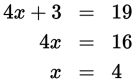# SAT Math Multiple Choice Question 515: Answer and Explanation

### Test Information

Question: 515

5. If 4x + 3 = 19, what is the value of 4x – 3?

• A. –19
• B. 4
• C. 13
• D. 19

Explanation:

C

Difficulty: Easy

Category: Heart of Algebra / Linear Equations

Strategic Advice: Don't let this fairly simple question fool you. Just because 3 and –3 are opposites, this does not mean the value on the right-hand side of the equals sign will be the opposite of 19.

Getting to the Answer: Solve for x, then substitute that value into the second equation for x and simplify.Thus, 4x – 3 = 4(4) – 3 = 16 – 3 = 13.

You might also recognize that 4x – 3 is 6 less than 4x + 3, so you can simply subtract 6 from 19 to arrive at 13.